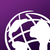# Email format

244
1
04-18-2020 01:08 PMNew Contributor II

I am trying to ask the data entry users to enter an email with the below format

`regex(., '^(([^<>()\[\]\\.,;:\s@"]+(\.[^<>()\[\]\\.,;:\s@"]+)*)|(".+"))@((\[[0-9]{1,3}\.[0-9]{1,3}\.[0-9]{1,3}\.[0-9]{1,3}])|(([a-zA-Z\-0-9]+\.)+[a-zA-Z]{2,}))\$')`

My question is what is the type should be and also where I should put the regex function. Is it under constraint.by